Advances in Wireless Communications and Networks
Volume 1, Issue 3, September 2015, Pages: 17-20

Time Varying Communication Channel Estimation Using Kalman Filters

Korhan Cengiz

Electrical-Electronics Engineering Department, Engineering Faculty, Trakya University, Edirne, Turkey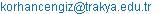Korhan Cengiz. Time Varying Communication Channel Estimation Using Kalman Filters.Advances in Wireless Communications and Networks.Vol.1, No. 3, 2015, pp. 17-20. doi: 10.11648/j.awcn.20150103.11

Abstract: In this study, time varying channel estimation problem was realized by using Kalman Filters. In the first part of the study, the introduction and some definitions were given. In the second part, the problem was analyzed and some useful theoretical and practical informations were given. In the third part of the study, the method Kalman Filters were explained and the simulation algorithm was given. In the last part of the study the simulation results were given and these results were explained and commented.

Keywords: Kalman Filters, Communication Channels, Channel Estimation

Contents

1. Introduction

1.1. Definitions

Channel: In its most General sense can describe everything from the source to the sink of the radio signal. Including the physical medium.

Channel Model: Is a mathematical representation of the transfer characteristics of the physical medium.

Channel models are formulated by observing the characteristics of the received signal.

The one that best explains the received signal behavior is used to model the channel.

Channel Estimation: The process of characterizing the effect of the physical medium on the input sequence.

1.2. General Channel Estimation ProcedureFigure 1. General Channel Estimation Procedure.

Aim of any channel estimation procedure:

Minimize some sort of criteria, e.g. MSE.

Utilize as little computational resources as possible allowing easy implementation.

Why Channel Estimation?

Allows to receiver to approximate effect of the channel on the signal.

The channel estimate is essential for removing inter symbol interference, noise rejection techniques etc.

Also used in diversity combining, ML detection, angle of arrival estimation etc.

1.3. Defining the Problem

Many transmission channels can be characterized as being linear but not time invariant. These are referred to by various names such as fading dispersive channels or fading multipath channels.

If we sample the output of the channel, then it can be shown that a good model is the low-pass tapped delay line model.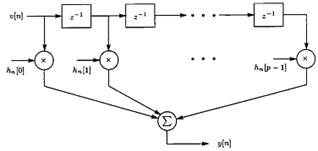Figure 2. Tapped delay line channel model.

The input-output description of this system is: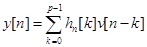(1)

This is really nothing more than an FIR filter with time – varying coefficients. To design effective communication or sonar systems it is necessary to have knowledge of these coefficients.

2. Problem

The problem becomes one of estimating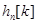based on the noise corrupted output of the channel.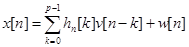(2)

Where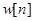is observation noise.

The linear model could be applied to estimate the deterministic parameters. In linear model the filter coefficients were time invariant. So, the linear model is not enough for this problem because there are too many parameters to estimate and the coefficients are time varying so we need KALMAN FILTERS.

To see more clearly why we use Kalman, let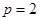and assume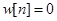for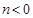The observations are, from (2),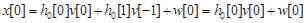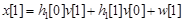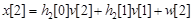Without corrupting noise we can not determine the tapped delay line weights. A way to solve this problem is to realize that the weights will not change rapidly from sample to sample, as an example, in a slow fading channel. For example in figure 2 this would correspond to an amplitude modulation which is slow.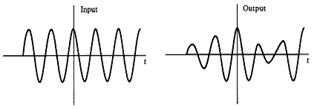Statistically, we may interpret the slow variation as a high degree of correlation between samples of the same tap weight.

This observation naturally leads us to model the tap weights as random variables whose time variation is described by a Gauss – Markov model.

Hence, we suppose that the state vector is(3)

Where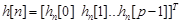,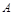is known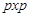matrix, and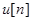is vector WGN with covariance matrix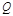.

To simplify the modeling the uncorrelated scattering can be used. It assumes that the tap weights are uncorrelated with each other and hence independent due to the jointly Gaussian assumption. As a result, we can assume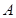,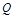and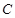the covariance matrix of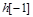, be diagonal matrices.

The vector Gauss – Markov model then becomes p independent scalar models. The measurement model becomes, from (2),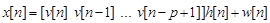whereis assumed to be WGN with variance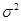and the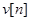sequence is assumed to known (since it is provided the input to the channel).

3. Kalman Filters

We can now form the MMSE estimator for the tapped delay line weights recursively in time using the Kalman Filter equations for a vector state and scalar observations.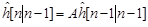(a)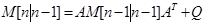(b)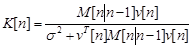(c)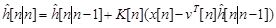(d)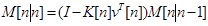(e)

(a): Prediction.

(b): Minimum Prediction MSE.

(c): Kalman Gain.

(d): Correction.

(e): Minimum MSE.

4. Simulations

The Kalman equations are initialized by,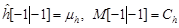.

We implemented the Kalman Filter estimator for a tapped delay line havingweights. We assume a state model with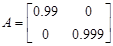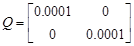Simulation Results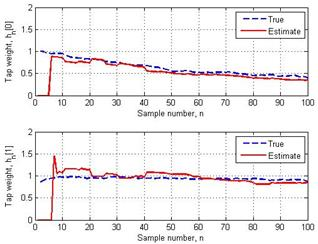Figure 4. Realization of TDL Coefficients.

A particular realization is shown in figure 4. In which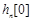is decaying to zero while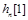is fairly constant. This is because the mean of the weights will be zero in steady – state. Due to the smaller value of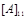,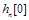will decay more rapidly.

Also, note that the eigenvalues of A are just the diagonal elements and they are less than 1 in magnitude. For this tap weight realization and the input shown in first part of figure 5, the noiseless output is shown in second part of figure 5 as determined from (1). When observation noise is added with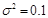, we obtain channel output shown in third part of figure 5.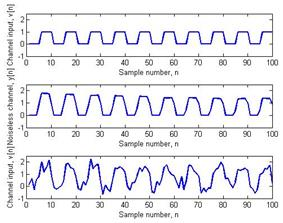Figure 5. Input – output waveforms of channel.

The Kalman filter is applied with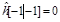and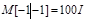, which were chosen to reflect little knowledge about the initial state. In the theoretical development of the Kalman filter the estimate of the initial state is given by the mean of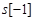. In practice this is seldom known, so that we usually just choose an arbitrary initial state estimate with a large initial MSE matrix to avoid ‘biasing’ the Kalman filter towards that assumed state. The estimated tap weights are shown in figure 6. After an initial transient, the Kalman filter locks on to the true weights and tracks them closely.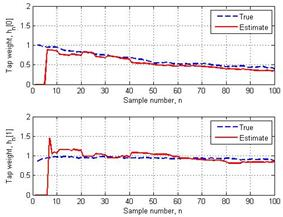Figure 6. Kalman filter estimate.

The Kalman filter gains are shown in figure 7. They appear to attain a periodic steady – state, although this behavior is different than the usual steady – state which is discussed previously sincevaries with time and real steady – state is never attained. Also, at times the gain is zero, as for example in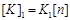for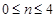. This is because at these timesis zero due to the zero input and thus the observations contain only noise. The Kalman filter ignores these data samples by forcing the gain to be zero.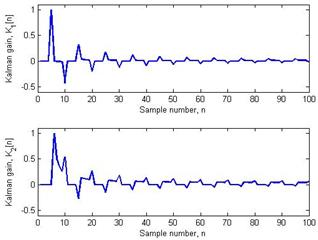Figure 7. Kalman filter gains.

The minimum MSEs are shown in figure 8 and are seen to decrease monotonically, although this generally will not be the case for a Kalman filter.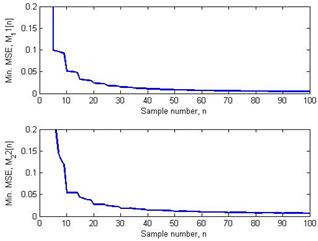Figure 8. Kalman filter minimum MSE.

5. Conclusion

In this paper, some definitions are given, then the problem statement was explained, the method Kalman Filters and its algorithm was given. Finally the simulation results were given and they are commended.

As a conclusion, in time varying coefficients, we can not use linear model, we need Kalman Filters because in this problem it is not possible to extend linear approach to this problem since there are too many parameters to estimate. We assumed the filter coefficients are time invariant. Consequently, the linear model could be applied to estimate the deterministic parameters.

In this paper, the channel is modeled as an FIR filter with time varying coefficients. The observation model is assumed to be Gauss-Markov for tap weights. Kalman filter is used to estimate the time varying coefficients of the channel.

References

1. Fundamentals of Statistical Signal Processing: Estimation Theory by Steven M. Kay (ISBN 0-13-345711-7).
2. Mathematical Statistics and Data Analysis by John Rice. (ISBN 0-534-209343).
3. An Introduction to Signal Detection and Estimation by H. Vincent Poor (ISBN 0-387-94173-8).
4. Detection, Estimation, and Modulation Theory, Part 1 by Harry L. Van Trees (ISBN 0-471-09517-6; website).
5. Optimal State Estimation: Kalman, H-infinity, and Nonlinear Approaches by Dan Simon website.
6. Ali H. Sayed, Adaptive Filters, Wiley, NJ, 2008, ISBN 978-0-470-25388-5.
7. Ali H. Sayed, Fundamentals of Adaptive Filtering, Wiley, NJ, 2003, ISBN 0-471-46126-1.
8. Thomas Kailath, Ali H. Sayed, and Babak Hassibi, Linear Estimation, Prentice-Hall, NJ, 2000, ISBN 978-0-13-022464-4.
9. Babak Hassibi, Ali H. Sayed, and Thomas Kailath, Indefinite Quadratic Estimation and Control: A Unified Approach to H2 and Hoo Theories, Society for Industrial & Applied Mathematics (SIAM), PA, 1999, ISBN 978-0-89871-411-1.
10. V.G.Voinov, M.S.Nikulin, "Unbiased estimators and their applications. Vol.1: Univariate case", Kluwer Academic Publishers, 1993, ISBN 0-7923-2382-3.
11. V.G.Voinov, M.S.Nikulin, "Unbiased estimators and their applications. Vol.2: Multivariate case", Kluwer Academic Publishers, 1996, ISBN 0-7923-3939-8.

 Contents 1. 1.1. 1.2. 1.3. 2. 3. 4. 5.
Article ToolsAbstractPDF(310K)